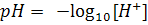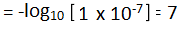Determine pH with pH indicator strips / universal indicator solution

# Our Objective

To find the pH of the following samples by using pH paper / universal indicator.

1. Dilute hydrochloric acid.
2. Dilute NaOH solution.
3. Dilute ethanoic acid solution.
4. Lemon juice.
5. Water.
6. Dilute sodium bicarbonate solution.

# The Theory

## Can you define pH?

pH is defined as the negative logarithm(base 10) of the hydrogen ion concentration in moles per litre. pH is written as:Hydrogen ions cannot exist alone, but they exist after combining with water molecules. Thus, H+must always be shown as H+(aq) or hydronium ion(H3O+):## How do we represent the pH of a neutral solution?Neutral solution has pH value 7. pH of acid solution is always less than 7,  whereas that of alkaline solution is always more than 7. pH of pure water is 7 or [H+]=10-7 mol/L .

## What is a pH indicator?

pH indicator is a chemical that turns different colours in different media. For example, blue litmus turns red in acidic medium, the red litmus turns blue in alkaline medium.

## What is a litmus solution?

Litmus solution is a purple dye, which is extracted from a Thallophyte known as Lichen. Litmus solution when in neutral medium (i.e., neither acidic nor basic) is greenish in colour.

## What are the commonly used indicators to determine pH of a solution?

Litmus solution and litmus paper are commonly used as an indicator. pH paper is a piece or a strip of paper which is coated with pH-indicator.

## Know what a pH scale is?

A scale for measuring hydrogen ion concentration is known as pH scale. In the term pH, p stands for 'potenz' in German meaning power.# Learning Outcomes

1. Students understand the terms pH, acidic, basic and  neutral through the experiments.
2. Students acquire the following skills after performing the experiment.
• How to measure the pH of a given solution using pH paper or universal indicator solution.
• How to classify the sample as acidic, basic or neutral based on the pH value.
• How to correlate the values obtained from the experiment with the pH scale

Cite this Simulator: